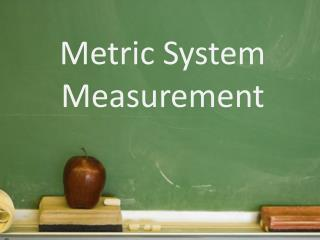DownloadDownload PresentationMetric System Measurement

# Metric System Measurement

Télécharger la présentation## Metric System Measurement

- - - - - - - - - - - - - - - - - - - - - - - - - - - E N D - - - - - - - - - - - - - - - - - - - - - - - - - - -
##### Presentation Transcript

1. Metric System Measurement

2. Bell Ringer • The Metric Conversion Act of 1975 determined that the metric system would be the system of measurement used for US Trade & Commerce. Why do you believe this act was passed?

3. History of the Metric System • After the French Revolution, Louis XVI put a group of scientists to create a unified system of measurement. • The SI System gives credit to the French because it stands for “Systeme International d’unites”

4. Length • Length is the distance between 2 objects • A meter stick measures length

5. Mass • Amount of matter in an object • Mass is measured with a triple beam balance • Grams is the basic unit of mass • Weight is not Mass because weight is affected by gravity.

6. Volume • Is the amount of space an object occupies • If it’s a solid = cm3. If it’s a liquid= mL • Solid= Calculate LxWxH • Liquid= Grad. Cylinder • Practice Problem:

7. Today’s Bell Ringer By saying a particular substance is more dense or less dense, what does this imply? Write down an example of two substances and compare their densities.

8. Density • Heaviness of objects. Compare a rock to a crumbled piece of paper. The rock is more dense than the paper. • Formula for Density = Mass/Volume • Practice Problem: Calculate the density in g/mL of aluminum if a 50 mL block weighs135 g.

9. Temperature • Measure of the average kinetic energy of the particles in a sample of matter • Measured in Celsius, Use a thermometer • Therm= heat, meter= measure • Convert 75 degrees Fahrenheit to Celsius • 5/9(degrees in Fahrenheit – 32)

10. Conversion Factor • Start with what’s given • Find a conversion factor • Numerator (unit that matches answer) • Denominator (unit that matches given #) • Multiply Across and then divide

11. CW/HW: RQ SI System Part 2 1. The unit kilograms implies we are measuring? 2. Cm3 implies we are measuring the volume of a ____? 3. Measure the volume of your desk: • Length b. Width c. Height 4. Greater mass? 45.7mL of a liquid or 27.8 mL of that same liquid